# MCQs on Electric Circuits

##### Page 56 of 63. Go to page 1 2 3 4 5 6 7 8 9 10 11 12 13 14 15 16 17 18 19 20 21 22 23 24 25 26 27 28 29 30 31 32 33 34 35 36 37 38 39 40 41 42 43 44 45 46 47 48 49 50 51 52 53 54 55 56 57 58 59 60 61 62 63
01․ A constant current source supplies a electric current of 300 mA to a load of 1 KΩ. When the load changed to 100 Ω, the load current will be
3 A.
300 mA.
30 mA.
100 mA.

As it is a constant current source, the value of the current will remain same whatever be the load.

02․ A voltage source having an open circuit voltage of 100 V and internal resistance of 50 Ω is equivalent to a current source
2 A with 50 Ω in series.
2 A with 50 Ω in parallel.
0.5 A in parallel with 50 Ω.
none of these.

The value of current source is 100/50 = 2A and the value of the resistance remains same.

03․ Current source has internal impedance connected in parallel with it whereas voltage source has internal impedance connected in __________________ with it.
series
parallel
both of above
none of them

Internal resistances are always connected in series with a voltage source.

04․ An ideal constant voltage source has _______________ internal impedance whereas a constant current source has ____________ internal impedance.
infinite, zero
zero, zero
zero, infinite
infinite, infinite

When an ideal voltage source has zero internal resistance, it can drop all of its voltage perfectly across a load in a circuit. No power is wasted due to zero internal resistance. The ideal voltage source can efficiently drop all of its voltage across a load. When the current source has infinite internal resistance, the output current from the source will not change despite changes in resistance value from the load.

05․ i) In star connection line current is equal to phase current. ii) In delta connection line voltage is equal to phase voltage. For a given phase connected to the particular line which of the statement is true ?
Only (1).
Only (2).
Both (1) and (2).
None of these.

In case of star connection, line current = phase current and line voltage = √3 × phase voltage . In case of delta connection, line voltage = phase voltage and line current = √3 × phase current.

06․ The delta equation of given star connected impedance ZQR is equal to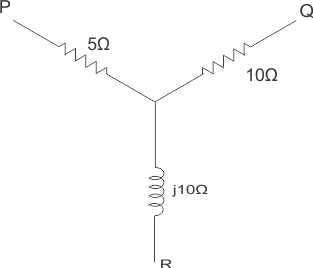40 Ω.
(20 + j10) Ω.
(5 + j10 / 3) Ω.
(10 + j30) Ω.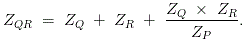07․ A star connected load has three equal impedance each of (40 + j30) Ω If the line current is 5 A then value of line voltage is
250 V.
250 √3 V.
250 / √3 V.
200 V.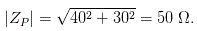Phase Voltage VP = IL × ZP. Line Voltage VL = √3 × VP.

08․ A delta connection contains three equal impedance of 60 Ω. The impedance of the equivalent star connection for each star load will be
15 Ω.
20 Ω.
30 Ω.
40 Ω.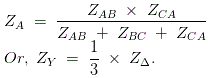09․ A balanced star connected load with impedance of 30j - 300 Ω is supplied from a 3 - phase, 4-wire, 173 V system, the voltages to neutral being 100j -900, 100j - 300 and 100j -1500 V. The current in neutral wire is
zero.
5 A.
8.85 A.
50 A.

Since the applied 3 - phase voltage is balanced and the impedance is all equal, so the current also would be balanced. As a result, there is no current in the neutral wire.

10․ A balanced delta connected load has an impedance of 9∠30o Ω per phase. What is the impedance per phase of its equivalent star?
27∠30o Ω
30∠30o Ω.
3∠30o Ω.
None of the above.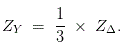<<<5455565758>>>